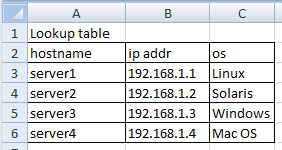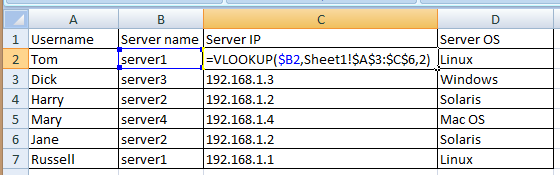# Software >> Applications >> Microsoft Office >> Excel >> How to use vlookup function populate columns by looking up a reference (lookup) table in another worksheet of the same workbookFig. 1  The lookup table is in Sheet1

The range to use as lookup is \$A:\$C.    \$ is used to make the it an absolute reference (does not change when copying to other cells)Fig 2  The table with columns to be populated is in another worksheet.  Use 'worksheetname'! to prefix the range to refer to different sheet.

VLOOKUP(lookup_value,table_array,col_index_num,[range_lookup])

lookup_value : the cell reference of containing the value to use as the key for the lookup

table_array : the range of cells that form the lookup table, with the lookup value in the first column and starting from first row

col_index_num : the number of columns relative to the lookup column

[range_lookup]